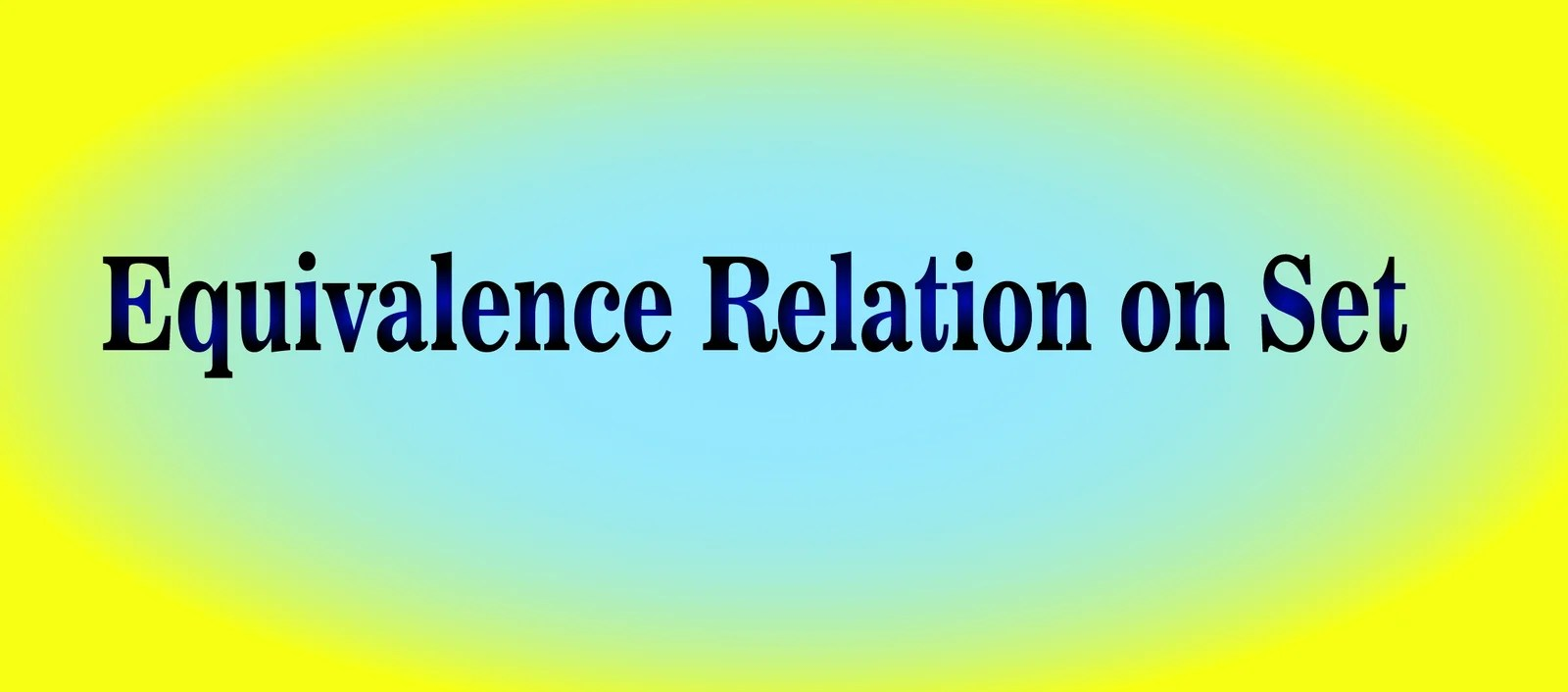# Equivalence Relation on Set (advance math)# Equivalence Relation on Set

Equivalence
relation on set is a relation which is reflexive, symmetric and transitive.

A relation
R, defined in a set A, is said to be an equivalence relation if and only if

(i) R is
reflexive, that is, aRa for all a ∈ A.

(ii) R is symmetric, that is, aRb ⇒ bRa for all a, b ∈ A.

(iii) R is transitive, that is aRb and bRc ⇒ aRc for all a, b, c ∈ A.

The
relation defined by “x is equal to y” in the set A of real numbers is an
equivalence relation.

Let A be a set of triangles in a plane. The relation R is defined as “x is similar to y, x, y ∈ A”.

We see
that R is;

(i)
Reflexive, for, every triangle is similar to itself.

(ii)
Symmetric, for, if x be similar to y, then y is also similar to x.

(iii)
Transitive, for, if x be similar to y and y be similar to z, then x is also
similar to z.

Hence R is
an equivalence relation.

A relation
R in a set S is called a partial order relation if it satisfies the following
conditions:

(i) aRa
for all a∈ A,
[Reflexivity]

(ii) aRb
and bRa ⇒ a =
b, [Anti-symmetry]

(iii) aRb and bRc ⇒ aRc, [Transitivity]

In the set
of natural numbers, the relation R defined by “aRb if a divides b” is a partial
order relation, since here R is reflexive, anti-symmetric and transitive.

A set, in
which a partial order relation is defined, is called a partially ordered set or
a poset.

Solved example on equivalence relation on set:

1. A relation R is defined on the set
Z by “a R b if a – b is divisible by 5” for a, b ∈ Z. Examine if R is an equivalence
relation on Z.

Solution:

(i) Let a ∈ Z. Then a – a is divisible by 5.
Therefore aRa holds for all a in Z and R is reflexive.

(ii) Let a, b ∈ Z and aRb hold. Then a – b is divisible by 5 and therefore b –
a is divisible by 5.

Thus, aRb ⇒ bRa and therefore R is symmetric.

(iii) Let a, b, c ∈ Z and aRb, bRc both hold. Then a
– b and b – c are both divisible by 5.

Therefore a – c = (a – b) + (b – c) is divisible by 5.

Thus, aRb and bRc  ⇒ aRc and therefore R is transitive.

Since R is
reflexive, symmetric and transitive so, R is an equivalence relation on Z.

2. Let m e a positive integer. A relation R is defined on the set Z by “aRb if and only if a – b is divisible by m” for a, b ∈ Z. Show that R is an equivalence relation on set Z.

Solution:

(i) Let a ∈ Z. Then a – a = 0, which is divisible by m

Therefore, aRa holds for all a ∈ Z.

Hence, R is reflexive.

(ii) Let a, b ∈ Z and aRb holds. Then a – b is divisible by m and therefore, b – a is also divisible by m.

Thus, aRb ⇒ bRa.

Hence, R is symmetric.

(iii) Let a, b, c ∈ Z and aRb, bRc both hold. Then a – b is divisible by m and b – c is also divisible by m. Therefore, a – c = (a – b) + (b – c) is divisible by m.

Thus,  aRb and bRc ⇒ aRc

Therefore, R is transitive.

Since, R is reflexive, symmetric and transitive so, R is an equivalence relation on set Z

3. Let S be the set of all lines in 3-dimensional space. A relation ρ is defined on S by “lρm if and only if l lies on the plane of m” for l, m ∈ S.

Examine if ρ is (i) reflexive, (ii) symmetric, (iii) transitive

Solution:

(i) Reflexive: Let l ∈ S. Then l is coplanar with itself.

Therefore, lρl holds for all l in S.

Hence, ρ is reflexive

(ii)  Symmetric: Let l, m ∈ S and lρm holds. Then l lies on the plane of m.

Therefore, m lies on the plane of l. Thus, lρm ⇒  mρl and therefore ρ is symmetric.

(iii) Transitive: Let l, m, p ∈ S and lρm, mρp both hold. Then l lies on the plane of m and m lies on the plane of p. This does not always implies that l lies on the plane of p.

That is, lρm and mρp do not necessarily imply lρp.

Therefore, ρ is not transitive.

Since R is reflexive and symmetric but not transitive so, R is not an equivalence relation on set Z

+